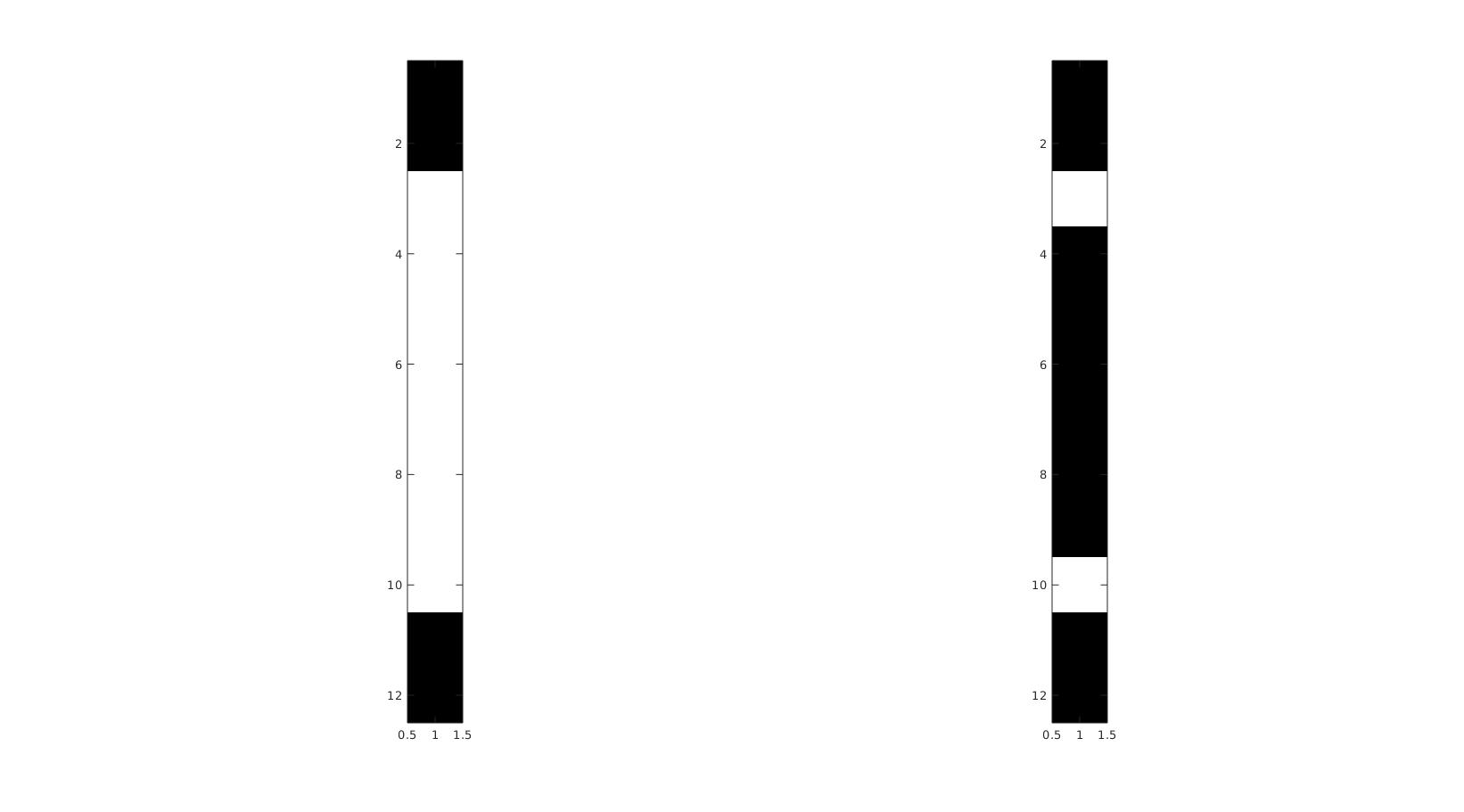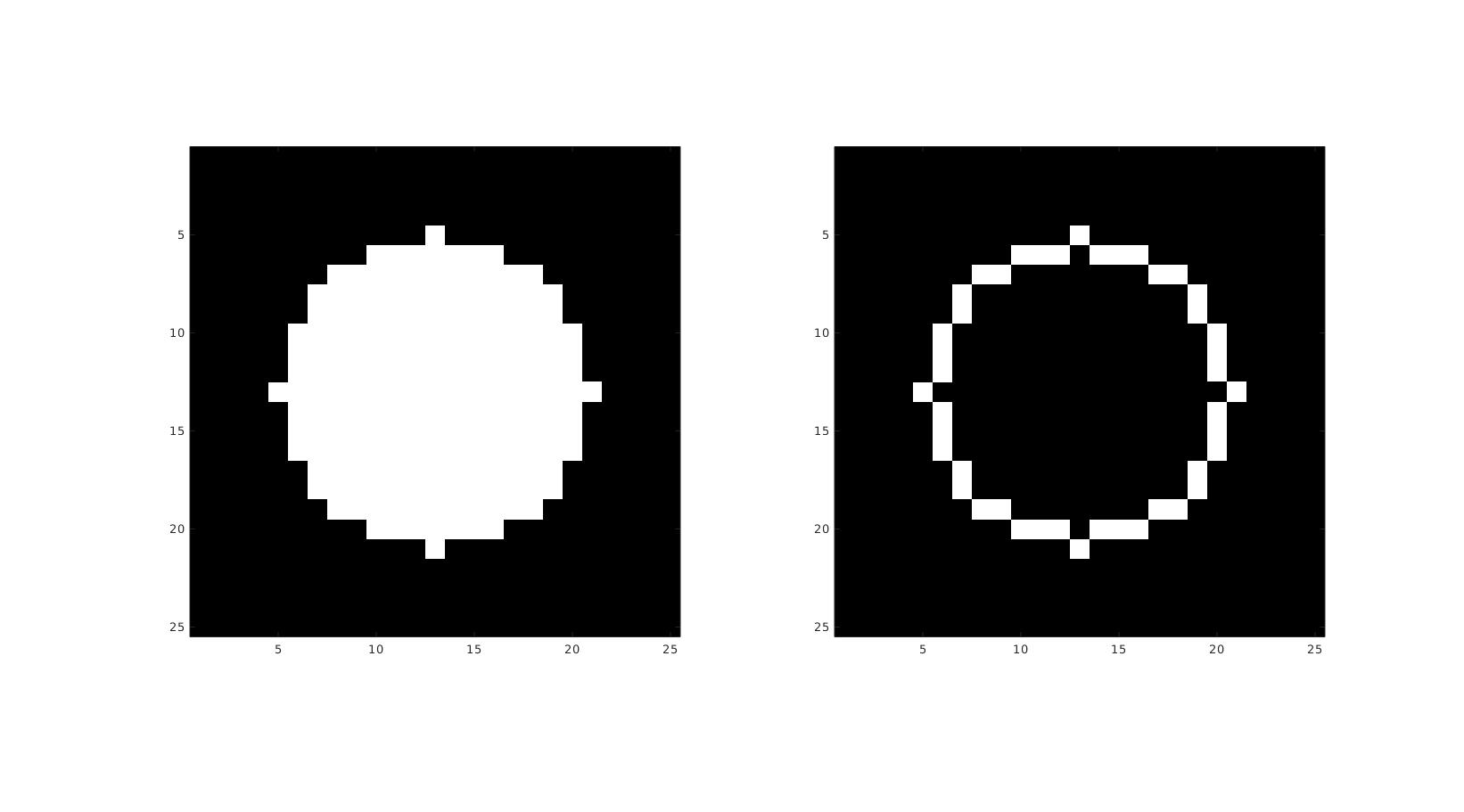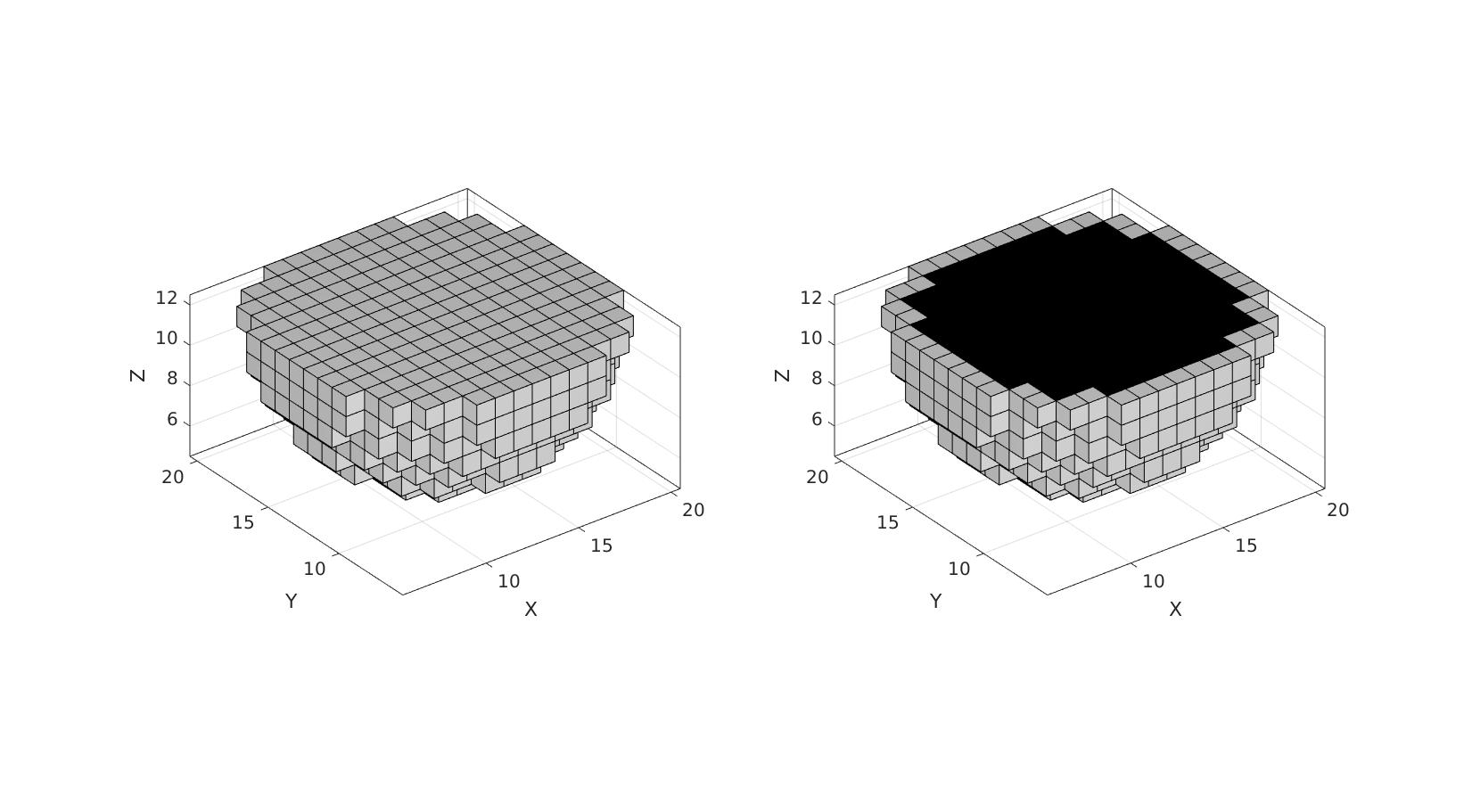# logicRemoveInterior

Below is a demonstration of the features of the logicRemoveInterior function

## Contents

```clear; close all; clc;
```

## Syntax

[Lb]=logicRemoveInterior(L);

## Description

This function removes the interior entries for the input logic L. Interior entries are those fully surrounded by neighbours (e.g. in 3D all entries which are attached to a top, bottom, left, right, front, and a back neighbour). Vectors and n-dimensional arrays are supported.

## Example 1: 1D logical treatment

```L = false(12,1);
L(3:10)=1;
```
```[Lb]=logicRemoveInterior(L);

[Lb]=logicRemoveInterior(L);
```
```cFigure;
subplot(1,2,1);
imagesc(L);
axis tight; axis equal; colormap gray;

subplot(1,2,2);
imagesc(Lb);
axis tight; axis equal; colormap gray;
drawnow;
cFigure;
subplot(1,2,1);
imagesc(L);
axis tight; axis equal; colormap gray;

subplot(1,2,2);
imagesc(Lb);
axis tight; axis equal; colormap gray;
drawnow;
```## Example 2: 2D logical treatment

```r=2;
n=25;
x=linspace(-1.5*r,1.5*r,n);
[X,Y]=ndgrid(x);
R = sqrt(X.^2+Y.^2);

L = R<=r;
```
```[Lb]=logicRemoveInterior(L);
```
```cFigure;
subplot(1,2,1);
imagesc(L);
axis tight; axis equal; colormap gray;

subplot(1,2,2);
imagesc(Lb);
axis tight; axis equal; colormap gray;
drawnow;
```## Example 3: 3D logical treatment

```r=2;
n=25;
x=linspace(-1.5*r,1.5*r,n);
[X,Y,Z]=ndgrid(x);
R = sqrt(X.^2+Y.^2+Z.^2);

L = R<=r;
```
```[Lb]=logicRemoveInterior(L);

%Force half to be zero so we can look inside
L(:,:,ceil(n/2):end)=0;
Lb(:,:,ceil(n/2):end)=0;
```
```[F,V,C]=im2patch(L,L);
[Fb,Vb,Cb]=im2patch(Lb,L);

cFigure;
subplot(1,2,1);
gpatch(F,V,C);
axisGeom; colormap gray; clim([0 1]);

subplot(1,2,2);
gpatch(Fb,Vb,Cb);
axisGeom; colormap gray; clim([0 1]);
drawnow;
```GIBBON www.gibboncode.org

Kevin Mattheus Moerman, [email protected]

GIBBON footer text

GIBBON: The Geometry and Image-based Bioengineering add-On. A toolbox for image segmentation, image-based modeling, meshing, and finite element analysis.

Copyright (C) 2006-2023 Kevin Mattheus Moerman and the GIBBON contributors

This program is free software: you can redistribute it and/or modify it under the terms of the GNU General Public License as published by the Free Software Foundation, either version 3 of the License, or (at your option) any later version.

This program is distributed in the hope that it will be useful, but WITHOUT ANY WARRANTY; without even the implied warranty of MERCHANTABILITY or FITNESS FOR A PARTICULAR PURPOSE. See the GNU General Public License for more details.

You should have received a copy of the GNU General Public License along with this program. If not, see http://www.gnu.org/licenses/.